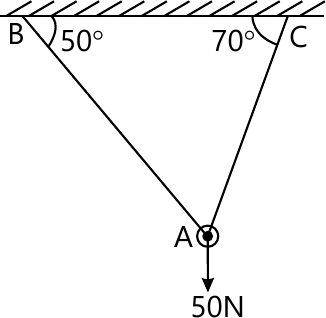# Engineering Mechanics Question paper May 2013

Engineering Mechanics Question paper May 2013 – The engineering mechanics E.M question paper for mumbai university students of semester 2 who are in F.E first year is given below:

### Engineering Mechanics Question paper May 2013

1 (a) Find forces P and Q such that resultant of given system is zero.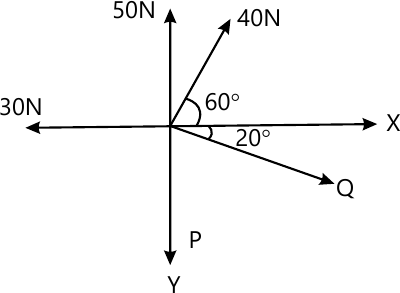4 M
1 (b) A cylinder B, WB=1000 N, dia 40 cm, hangs by cable AB=40 cm rests against a smooth wall. Find out reaction at C and TAB.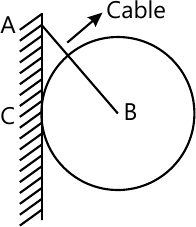4 M
1 (c) A block of weight 1000 N is kept on a rough inclined surface. Find out range of P for which the will block will be in equilibrium.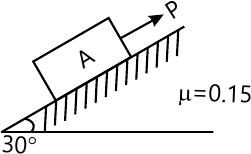4 M
1 (d) A curvilinear motion of a particle is defined by VX= 25 – 8t m/s and y=48 – 3t2m. At t=0, x=0, Find out position, velocity and acceleration at t=4 sec.
4 M
1 (e) State D’ Alembert’s principle with two examples.
4 M
2 (a) Find out resultant of given (lever) forces system w.r.t. “B”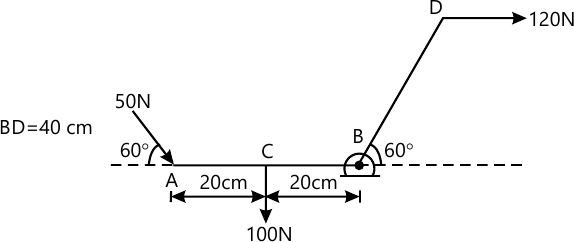6 M
2 (b) Two identical cylinders dia 100 mm weight 200 N are placed as shown. All contacts are smooth. Find out reaction at A,B and C.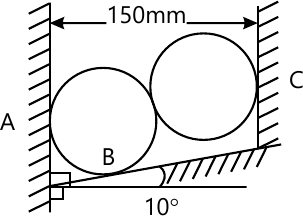8 M
2 (c) A ball of mass m kg hits an inclined smooth surface with a velocity VA=3 m/s. Find out velocity of rebound.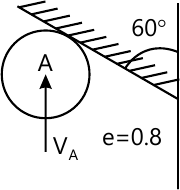6 M
3 (a) Find centoid of the shaded area.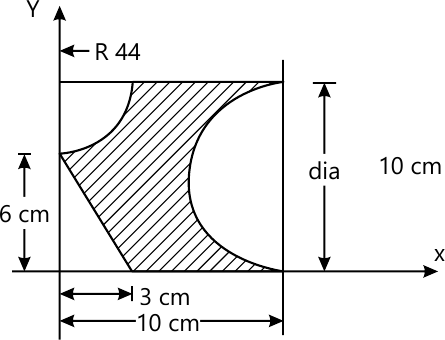8 M
3 (b) Explain conditions for equilibrium for forces in space.
6 M
3 (c) Explain work energy principle.
6 M
4 (a) Find the support reaction at Hinge A and Roller B.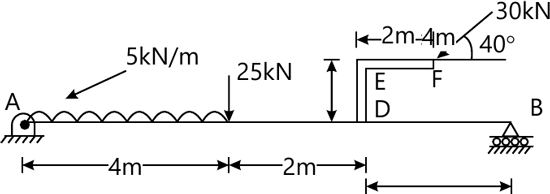8 M
4 (b) Explain x-t, v-t and a-t curves in Kinematics.
6 M
4 (c) Collar B moves up with velocity VB=2 m/s. Rod AB is pinned at B. Find out angular velocity of AB and velocity of A.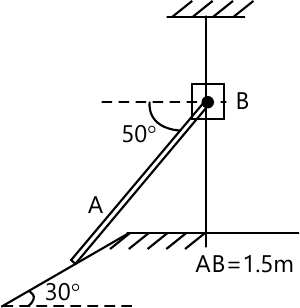6 M
5 (a) Find out forces in FB and BE using method of section and other members by method of joints.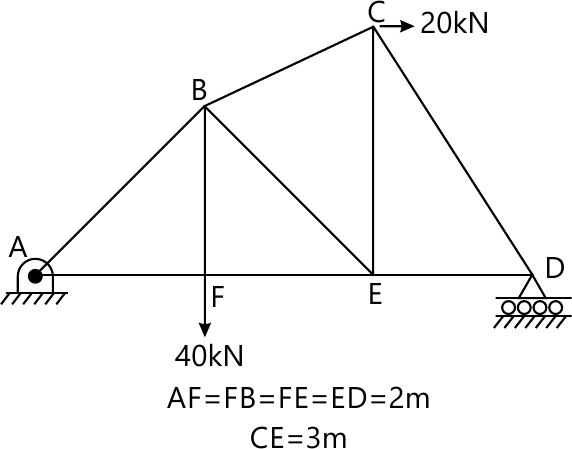8 M
5 (b) A stone is thrown vertically upwards and returns to the starting point at the ground in 6 sec. Find out max. height and initial velocity of stone.
6 M
5 (c) Explain instantaneous centre of rotation.
6 M
6 (a) Force F=(3j – 4j + 12k)N acts at point A(1, -2, 3). Find –
(i) Moment of force about origin
(ii) Moment of forces about point B(2,1,2)m
4 M
6 (b) Find out min, value of P to start the motion.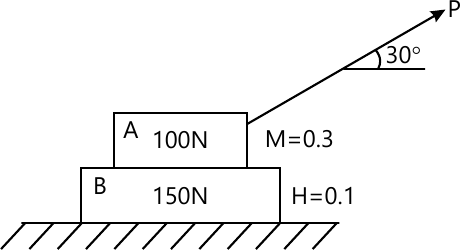8 M
6 (c) For a particle in rectilinear motion a= -0.05V2 m/s2, at v=20 m/s, x=0. Find x at v=15 m/s and accn at x=50 m.
4 M
6 (d) Sphere A is supported by two wires AB, AC. Find out tension in wire AC :-
(i) before AB is cut
(ii) just after AB is cut